### Geometry Puzzle: Center of Square in Circle

Source: Asked to me by a lot of people from IIT Bombay on email and posted by a few on "Post a Question" page.

Problem:
What is the probability that two uniform random points (to be precise: i.i.d. with respect to Lebesgue measure) in the square are such that center of the square lies in the circle formed by taking the points as diameter.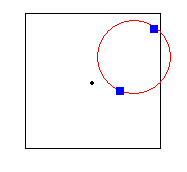Edit: Problem wording made more mathematical.

Update: (24/12/2012)
Correct solutions by Barun Kumar Kejriwal, Akhil Kumar, Aastha Airan, Arpit Goyal and Yashoteja in comments! Thanks a ton.

1.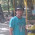Mark an arbitrary point P1 inside the square and draw a line L1 through P1 and the centre of the circle.
Draw another line L2 perpendicular to L1 and passing through the centre of the square, O.

Now, it becomes easy to see that any point in the portion of the square on the opposite side of L2 makes an obtuse angle with OP1.

Thus, prob = 1/2

2.(1/2)

Hint for people who want to try: Diametrically opposite points form obtuse angle at any point inside the circle, right angle at points on the circle and acute angle at the points outside the circle

3.The angle it the two points make with the center should be >= 90 (obtuse angle)
With half probability the angle will be obtuse
Probability = 1/2

4.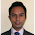Take one point inside the square. Join it to the centre of square. Now draw a line perpendicular to the given line. The perpendicular line divides the square into parts with equal area.

Now if I take the second point in the part which contains the first point, out points will subtend an acute angle at the centre.

If I take second point in the other half, our points will subtend an obtuse angle at the centre.

A point lies is circle obtained by two points as diameter if the two points subtend an obtuse angle.

So probability that centre lies inside the circle is same as probability that it lies outside the circle.

Ans: 0.5

5.Probability is 0.5

If 'O' is the center of the square,
and 'A' and 'B' are 2 points inside the square
chosen uniformly at random,

then O lies inside circle sitting on AB iff angle AOB is obtuse.

Now for any random point A inside the square,
placing B in some parts of square leads to acute angle, others to obtuse angle. By symmetry it is easy to see that both areas are equal, meaning probability of selecting A and B such that O lies inside the circle sitting on them is 0.5

(Draw lines 0A', OA", OC', OC" where,
A', A", C', C" lie on square and C'C" perp to A'A", and A lies on OA'. then area on one side of C'C" corresponds to acute angle, other to obtuse angle, both areas being equal)

6.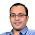Correct solutions by Barun Kumar Kejriwal, Akhil Kumar, Aastha Airan, Arpit Goyal and Yashoteja in comments! Thanks a ton.

7.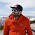One more variation might be what is the probability that circle formed lies completely inside the square :)